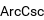# Get Tutoring Info Now!

(800) 434-2582

/ Get Math Help

## Inverse Cosecant

Alternate names
Definition

The inverse cosecant is the multivalued function csc^(-1) z, also denoted arccsc z, that is the inverse function of the cosecant. The variants Arccsc z (e.g., Beyer 1987, p. 141; Bronshtein and Semendyayev, 1997, p. 70) and Csc^(-1) z are sometimes used to refer to explicit principal values of the inverse cosecant, although this distinction is not always made (e.g, . Zwillinger 1995, p. 466). Worse yet, the notation arccsc z is sometimes used for the principal value, with Arccsc z being used for the multivalued function. Note that in the notation csc^(-1) z (commonly used in North America and in pocket calculators worldwide), csc z is the cosecant and the superscript -1 denotes an inverse function, not the multiplicative inverse. The principal value of the inverse cosecant is implemented as ArcCsc[x] in the Wolfram Language. The inverse cosecant is a multivalued function and hence requires a branch cut in the complex plane, which the Wolfram Language's convention places at (-1, 1). This follows from the definition of csc^(-1) z as csc^(-1) z = - i ln(sqrt(1 - 1/z^2) + i/z). The derivative of csc^(-1) z is given by d/(d z) csc^(-1) z = - 1/(z^2 sqrt(1 - 1/z^2)), which simplifies to d/(d x) csc^(-1) x = - 1/(x sqrt(x^2 - 1)) for x>0. Its indefinite integral is integral csc^(-1) z d z = z csc^(-1) z + ln[z(1 + sqrt((z^2 - 1)/z^2))] + C, which simplifies to integral csc^(-1) x d x = x csc^(-1) x + ln(x + sqrt(x^2 - 1)) for x>0. The inverse cosecant has Taylor series about infinity of csc^(-1) x | = | - sum_(n = 1)^∞ (i^(n + 1) P_(n - 1)(0))/n x^(-n) | = | (1/2)_(n - 1)/((n - 1)!(2n - 1)) x^(1 - 2n) | = | x^(-1) + 1/6 x^(-3) + 3/40 x^(-5) + 5/112 x^(-7) + ... (OEIS A055786 and A002595), where P_n(x) is a Legendre polynomial and (x)_n is a Pochhammer symbol.

Related terms
Related Wolfram Language symbol